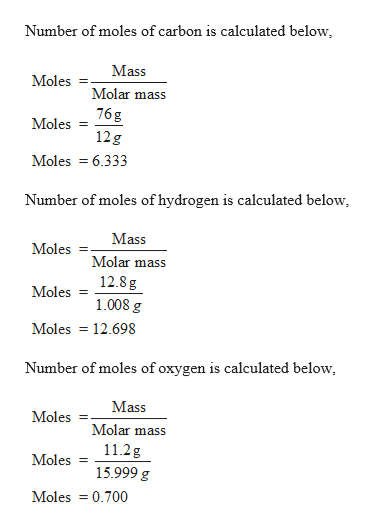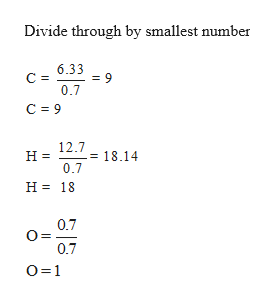# The percent composition by mass of a compound is 76.0% C, 12.8% H, and 11.2% O. The molar mass of this compound is 284.5 g/mol. What is the molecular formula of the compound?answer choicesC10H6OC9H18OC18H36O2C20H12O2C16H28O4

Question
146 views

The percent composition by mass of a compound is 76.0% C, 12.8% H, and 11.2% O. The molar mass of this compound is 284.5 g/mol. What is the molecular formula of the compound?

C10H6O

C9H18O

C18H36O2

C20H12O2

C16H28O4

check_circle

Step 1

Given,

The percent composition by mass of a compound is 76.0% C, 12.8% H, and 11.2% O.

The molar mass of this compound is 284.5 g/mol.

Molar mass of carbon = 12.0107

Molar mass of hydrogen = 1.008

Molar mass of oxygen = 15.999help_outlineImage TranscriptioncloseNumber of moles of carbon is calculated below, Mass Moles Molar mass 76 g Moles 12g Moles 6.333 Number of moles of hydrogen is calculated below, Mass Moles Molar mass 12.8 g Moles 1.008 g Moles 12.698 Number of moles of oxygen is calculated below Mass Moles = Molar mass 11.2g Moles 15.999 g Moles 0.700 fullscreen
Step 2

Therefore,

The ratio of the number of moles of carbon, hydroge...help_outlineImage TranscriptioncloseDivide through by smallest number 6.33 = 9 0.7 С - C 9 12.7 = 18.14 0.7 н Н3 18 0.7 0.7 O 1 fullscreen

### Want to see the full answer?

See Solution

#### Want to see this answer and more?

Solutions are written by subject experts who are available 24/7. Questions are typically answered within 1 hour.*

See Solution
*Response times may vary by subject and question.
Tagged in

### General Chemistry